# Lines and Slope Definition of Slope The slope

• Slides: 21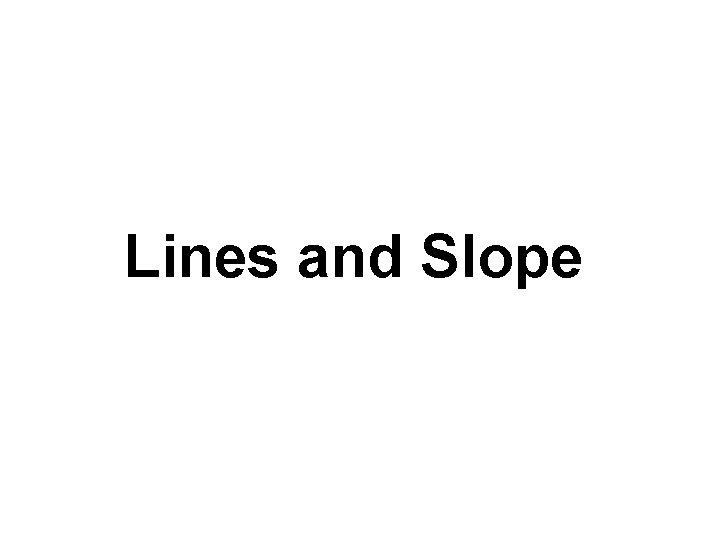Lines and Slope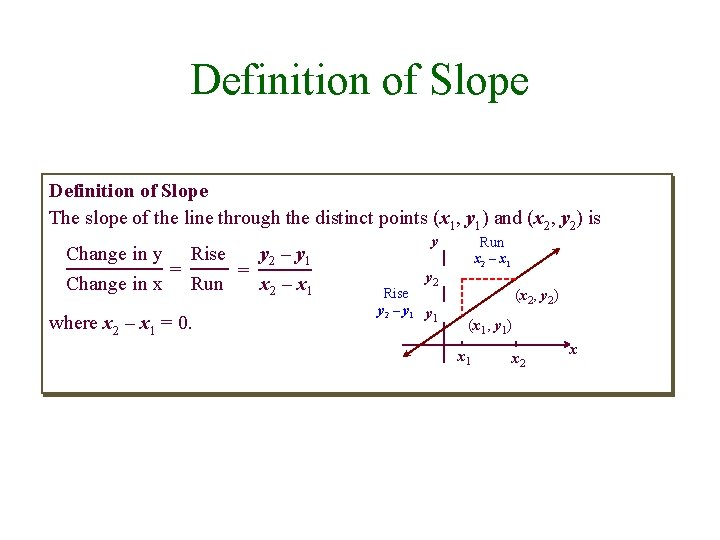Definition of Slope The slope of the line through the distinct points (x 1, y 1) and (x 2, y 2) is Change in y Rise y 2 – y 1 = = Change in x Run x 2 – x 1 where x 2 – x 1 = 0. y Run x 2 – x 1 y 2 Rise y 2 – y 1 (x 2, y 2) (x 1, y 1) x 1 x 2 x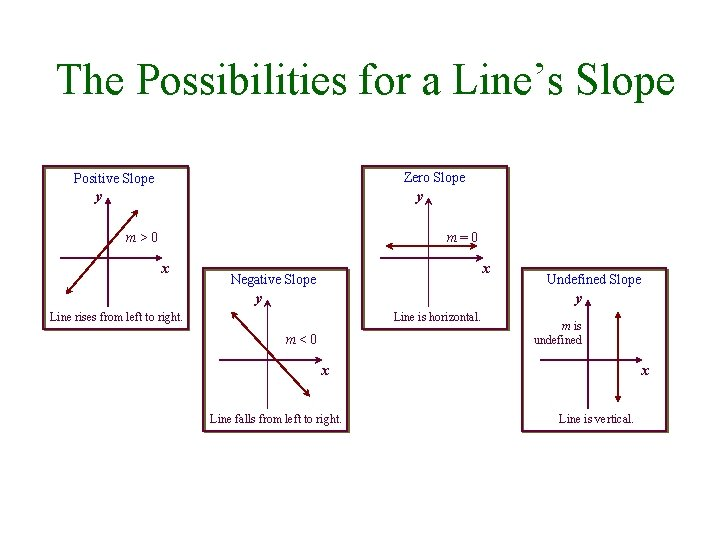The Possibilities for a Line’s Slope Zero Slope Positive Slope y y m>0 m=0 x x Negative Slope y Undefined Slope y Line rises from left to right. Line is horizontal. m<0 m is undefined x Line falls from left to right. x Line is vertical.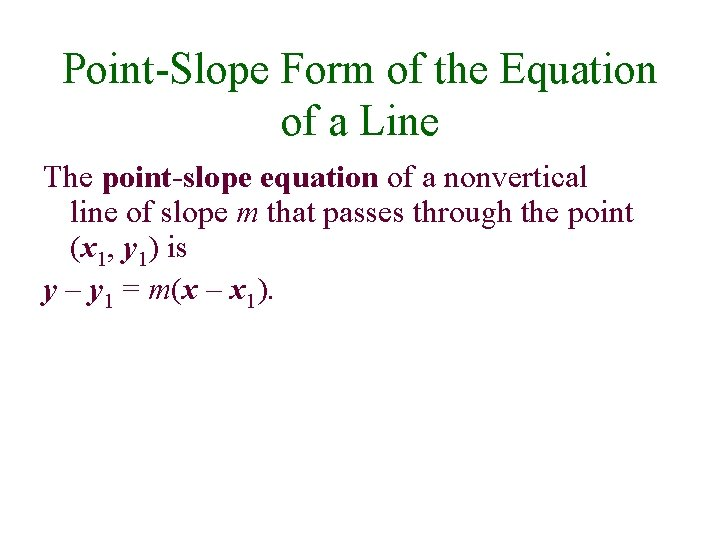Point-Slope Form of the Equation of a Line The point-slope equation of a nonvertical line of slope m that passes through the point (x 1, y 1) is y – y 1 = m(x – x 1).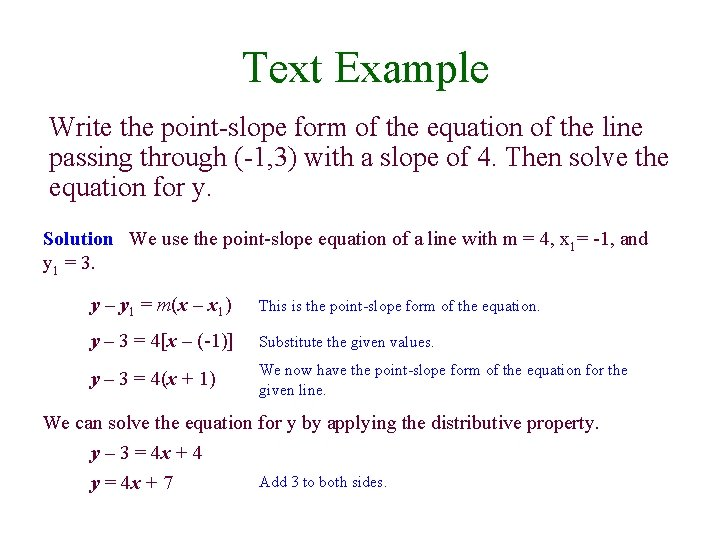Text Example Write the point-slope form of the equation of the line passing through (-1, 3) with a slope of 4. Then solve the equation for y. Solution We use the point-slope equation of a line with m = 4, x 1= -1, and y 1 = 3. y – y 1 = m(x – x 1) This is the point-slope form of the equation. y – 3 = 4[x – (-1)] Substitute the given values. y – 3 = 4(x + 1) We now have the point-slope form of the equation for the given line. We can solve the equation for y by applying the distributive property. y – 3 = 4 x + 4 Add 3 to both sides. y = 4 x + 7Slope-Intercept Form of the Equation of a Line The slope-intercept equation of a nonvertical line with slope m and y-intercept b is y = mx + b.Graphing y=mx+b Using the Slope and y-Intercept • Graphing y = mx + b by Using the Slope and y. Intercept • Plot the y-intercept on the y-axis. This is the point (0, b). • Obtain a second point using the slope, m. Write m as a fraction, and use rise over run starting at the yintercept to plot this point. • Use a straightedge to draw a line through the two points. Draw arrowheads at the ends of the line to show that the line continues indefinitely in both directions.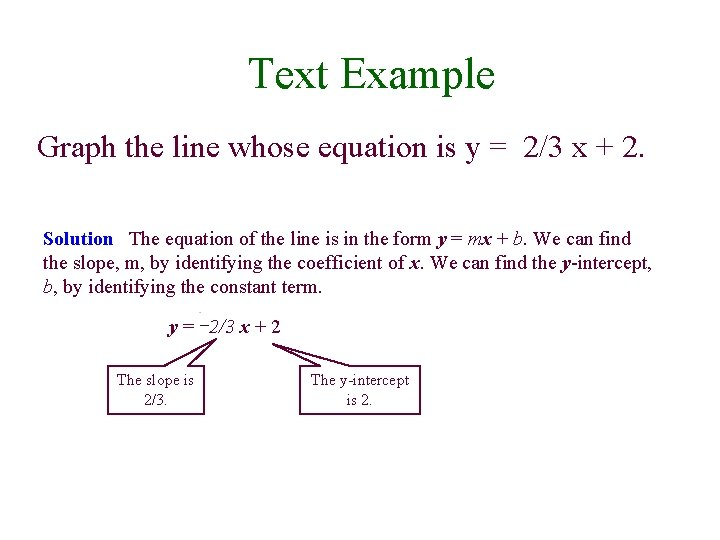Text Example Graph the line whose equation is y = 2/3 x + 2. Solution The equation of the line is in the form y = mx + b. We can find the slope, m, by identifying the coefficient of x. We can find the y-intercept, b, by identifying the constant term. y = 2/3 x + 2 The slope is 2/3. The y-intercept is 2.Text Example cont. Graph the line whose equation is y = 2/3 x + 2. Solution: We need two points in order to graph the line. We can use the yintercept, 2, to obtain the first point (0, 2). Plot this point on the y-axis. We plot the second point on the line by starting at (0, 2), the first point. Then move 2 units up (the rise) and 3 units to the right (the run). This gives us a second point at (3, 4). 5 4 3 2 1 -5 -4 -3 -2 -1 -1 -2 -3 -4 -5 1 2 3 4 5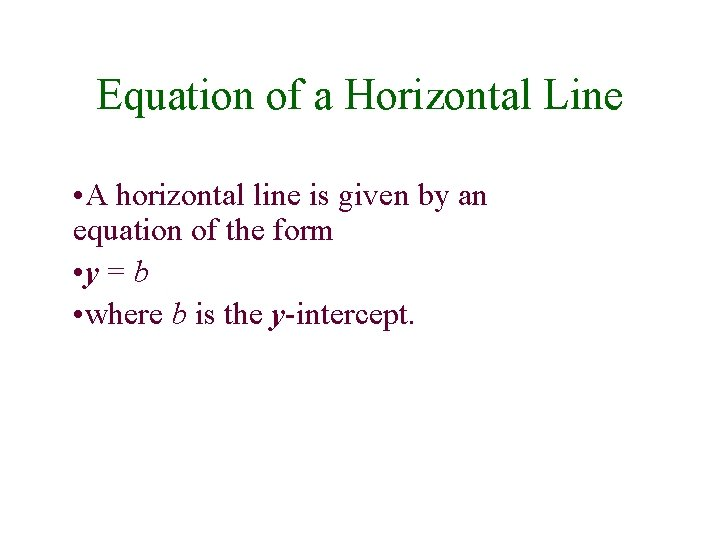Equation of a Horizontal Line • A horizontal line is given by an equation of the form • y = b • where b is the y-intercept.Equation of a Vertical Line • A vertical line is given by an equation of the form • x = a • where a is the x-intercept.General Form of the Equation of a Line Every line has an equation that can be written in the general form Ax + By + C = 0 Where A, B, and C are three real numbers, and A and B are not both zero.Text Example Find the slope and the y-intercept of the line whose equation is 2 x – 3 y + 6 = 0. Solution The equation is given in general form. We begin by rewriting it in the form y = mx + b. We need to solve for y. 2 x – 3 y + 6 = 0 This is the given equation. 2 x + 6 = 3 y To isolate the y-term, add 3 y on both sides. 3 y = 2 x + 6 Reverse the two sides. (This step is optional. ) y = 2/3 x + 2 Divide both sides by 3. The coefficient of x, 2/3, is the slope and the constant term, 2, is the y-intercept.Equations of Lines Point-slope form: y – y 1 = m(x – x 1) Slope-intercept form: y = m x + b Horizontal line: y = b Vertical line: x=a General form: Ax + By + C = 0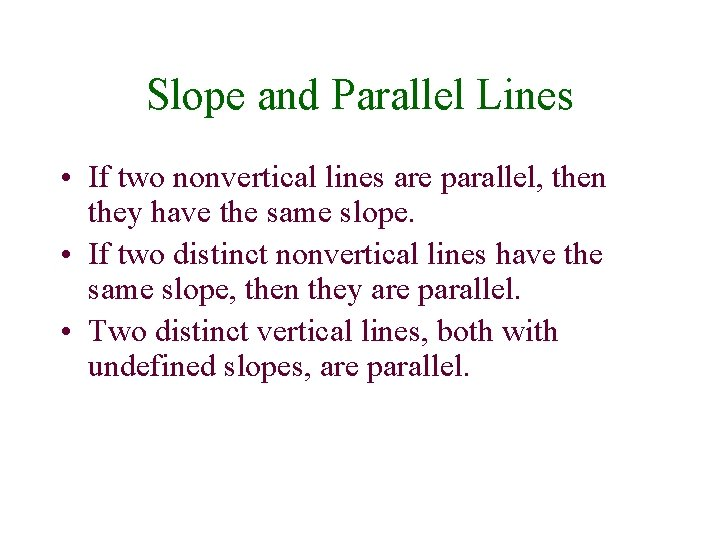Slope and Parallel Lines • If two nonvertical lines are parallel, then they have the same slope. • If two distinct nonvertical lines have the same slope, then they are parallel. • Two distinct vertical lines, both with undefined slopes, are parallel.Text Example Write an equation of the line passing through (-3, 2) and parallel to the line whose equation is y = 2 x + 1. Express the equation in point-slope form and yintercept form. Solution We are looking for the equation of the line shown on the left. Notice that the line passes through the point (-3, 2). Using the point-slope form of the line’s equation, we have x 1 = -3 and y 1 = 2. y – y 1 = m(x – x 1) 5 (-3, 2) y = 2 x + 1 4 3 Run = 1 2 y 1 = 2 x 1 = -3 1 -5 -4 -3 -2 -1 -1 -2 -3 -4 -5 Rise = 2 1 2 3 4 5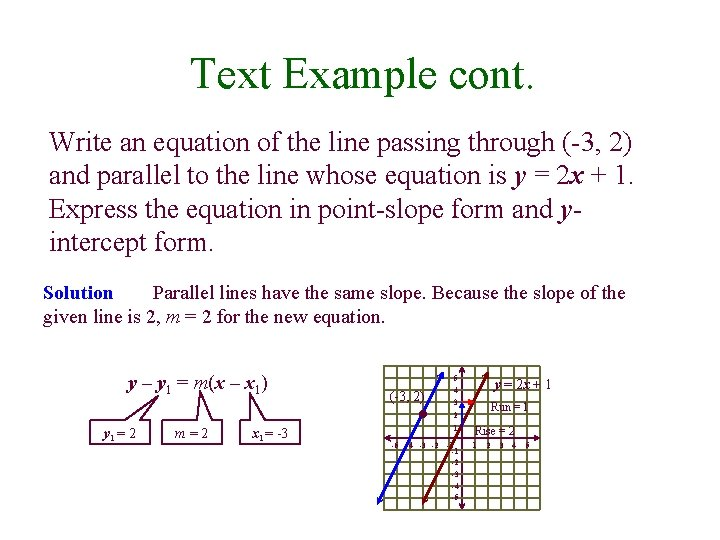Text Example cont. Write an equation of the line passing through (-3, 2) and parallel to the line whose equation is y = 2 x + 1. Express the equation in point-slope form and yintercept form. Solution Parallel lines have the same slope. Because the slope of the given line is 2, m = 2 for the new equation. y – y 1 = m(x – x 1) 5 (-3, 2) y = 2 x + 1 4 3 Run = 1 2 y 1 = 2 m=2 x 1 = -3 1 -5 -4 -3 -2 -1 -1 -2 -3 -4 -5 Rise = 2 1 2 3 4 5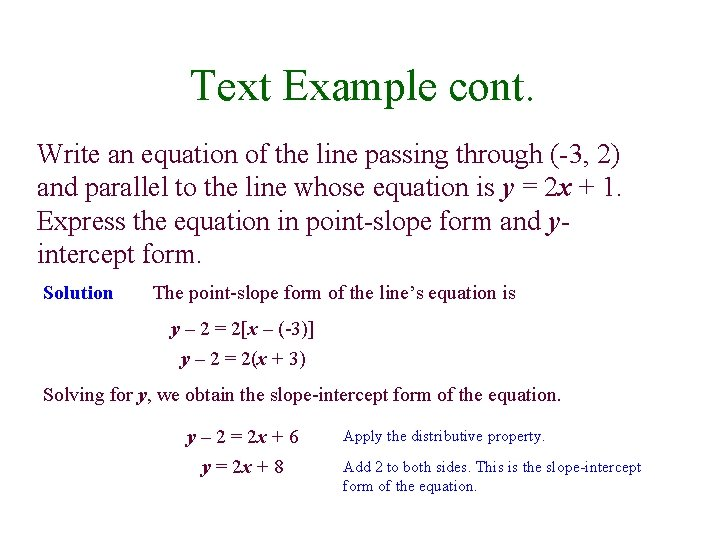Text Example cont. Write an equation of the line passing through (-3, 2) and parallel to the line whose equation is y = 2 x + 1. Express the equation in point-slope form and yintercept form. Solution The point-slope form of the line’s equation is y – 2 = 2[x – (-3)] y – 2 = 2(x + 3) Solving for y, we obtain the slope-intercept form of the equation. y – 2 = 2 x + 6 y = 2 x + 8 Apply the distributive property. Add 2 to both sides. This is the slope-intercept form of the equation.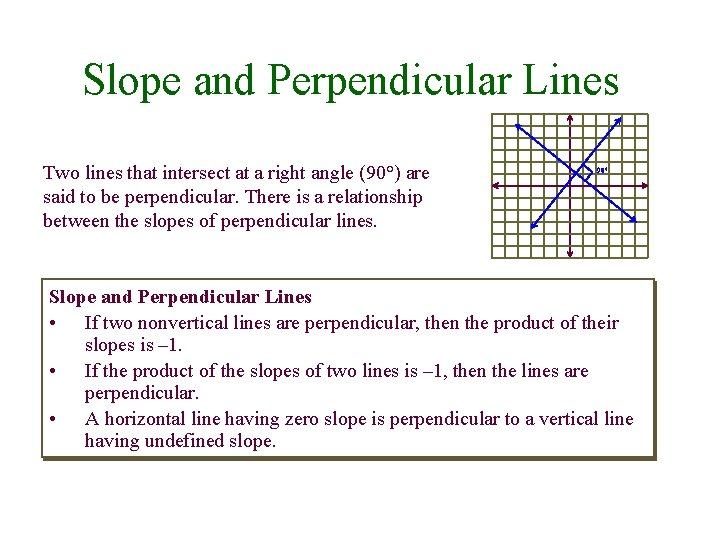Slope and Perpendicular Lines Two lines that intersect at a right angle (90°) are said to be perpendicular. There is a relationship between the slopes of perpendicular lines. 90° Slope and Perpendicular Lines • If two nonvertical lines are perpendicular, then the product of their slopes is – 1. • If the product of the slopes of two lines is – 1, then the lines are perpendicular. • A horizontal line having zero slope is perpendicular to a vertical line having undefined slope.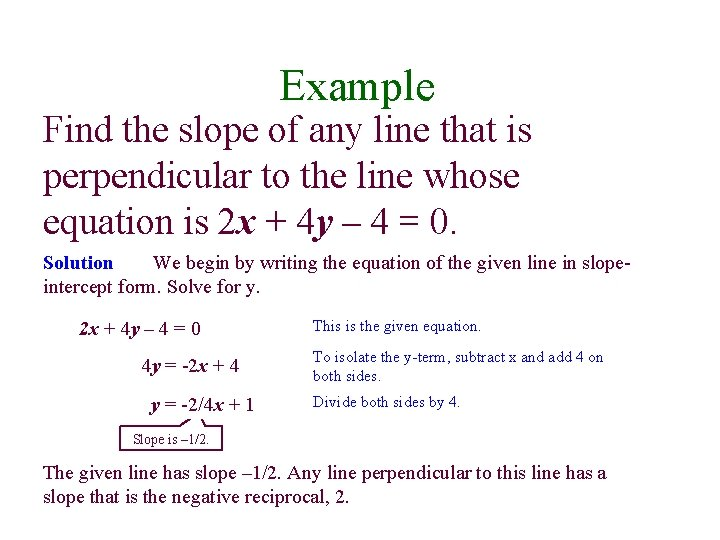Example Find the slope of any line that is perpendicular to the line whose equation is 2 x + 4 y – 4 = 0. Solution We begin by writing the equation of the given line in slopeintercept form. Solve for y. 2 x + 4 y – 4 = 0 4 y = -2 x + 4 y = -2/4 x + 1 This is the given equation. To isolate the y-term, subtract x and add 4 on both sides. Divide both sides by 4. Slope is – 1/2. The given line has slope – 1/2. Any line perpendicular to this line has a slope that is the negative reciprocal, 2.Lines and Slope